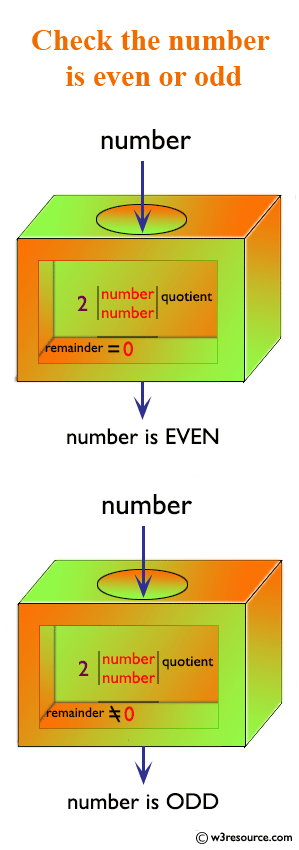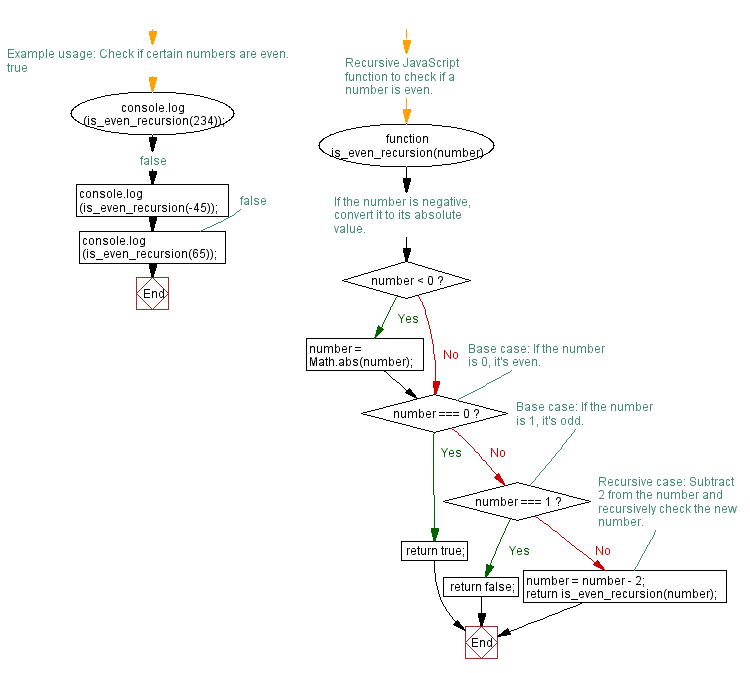# JavaScript: Check whether a number is even or not

## JavaScript Function: Exercise-7 with Solution

Write a JavaScript program to check whether a number is even or not.

Pictorial Presentation:Sample Solution-1:

Using recursion:

JavaScript Code:

``````function is_even_recursion(number)
{
if (number < 0)
{
number = Math.abs(number);
}
if (number===0)
{
return true;
}
if (number===1)
{
return false;
}
else
{
number = number - 2;
return is_even_recursion(number);
}
}
console.log(is_even_recursion(234)); //true
console.log(is_even_recursion(-45)); // false
console.log(is_even_recursion(65)); // false
```
```

Output:

```true
false
false
```

Flowchart:Sample Solution-2:

Without recursion:

Test even number:

• Checks whether a number is odd or even using the modulo (%) operator.
• Returns true if the number is even, false if the number is odd.

Test odd number:

• Check whether a number is odd or even using the modulo (%) operator.
• Return true if the number is odd, false if the number is even.

JavaScript Code:

``````const is_Even = num => num % 2 === 0;
const is_Odd = num => num % 2 === 1;
console.log("Test even number!")
console.log(is_Even(1));
console.log(is_Even(4));
console.log(is_Even(100));
console.log("Test odd number!")
console.log(is_Odd(1));
console.log(is_Odd(4));
console.log(is_Odd(107));
```
```

Output:

```Test even number!
false
true
true
Test odd number!
true
false
true
```

Live Demo:

See the Pen javascript-recursion-function-exercise-7 by w3resource (@w3resource) on CodePen.

Improve this sample solution and post your code through Disqus

What is the difficulty level of this exercise?

Test your Programming skills with w3resource's quiz.

﻿

## JavaScript: Tips of the Day

Log the values that are commented out after the console.log statement

```function* startGame() {
const answer = yield 'Do you love JavaScript?';
if (answer !== 'Yes') {
return "Oh wow... Guess we're gone here";
}
return 'JavaScript loves you back ?';
}

const game = startGame();
console.log(/* 1 */); // Do you love JavaScript?
console.log(/* 2 */); // JavaScript loves you back ?
```

A generator function "pauses" its execution when it sees the yield keyword. First, we have to let the function yield the string "Do you love JavaScript?", which can be done by calling game.next().value.
Every line is executed, until it finds the first yield keyword. There is a yield keyword on the first line within the function: the execution stops with the first yield! This means that the variable answer is not defined yet!
When we call game.next("Yes").value, the previous yield is replaced with the value of the parameters passed to the next() function, "Yes" in this case. The value of the variable answer is now equal to "Yes". The condition of the if-statement returns false, and JavaScript loves you back ? gets logged.

Ref: https://bit.ly/3jFRBje